# Algebra contest

This contest has ended on 3rd september

TOPICS

Relation and function, matrix and determinants, vector, system of equations, sequence and series, permutation and combination, binomial theorem, complex numbers, polynomials, inequalities .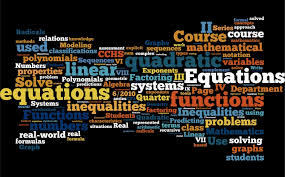Rules

1. I will post the first problem. If someone solves it, he or she can post a solution and then must post a new problem.

2. A solution must be posted below the thread of the problem. Then, the solver must post a new problem as a separate thread.

3. Please make a substantial comment.

4. Make sure you know how to solve your own problem before posting it, in case no one else is able to solve it within 24 hours. Then, you must post the solution and you have the right to post a new problem.

5. If the one who solves the last problem does not post a new problem in 2 hours, the creator of the previous problem has the right to post another problem.

6. It is NOT compulsory to post original problems. But make sure it has not been posted on Brilliant.

7. If a diagram is involved in your problem please make sure it is drawn by a computer program.

8. Format your solution in $\LaTeX$, picture solution will be accepted but only picture will not be, i.e. you can use picture for diagram or something, but not for the complete solution. Also make sure your solution is detailed and make sure to proof all claims.

9. Do not post problems on same topic frequently like 3 continuous inequality problem are not allowed. 2 are sufficient.

10. For those who are on slack Please post in #general that new problem is up along with problem number and the link to contest (https://brilliant.org/discussions/thread/algebra-contest/?sort=new)

11. Handwritten picture solution are accepted only when they are easily understandable!!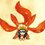Note by Prince Loomba
4 years, 11 months ago

This discussion board is a place to discuss our Daily Challenges and the math and science related to those challenges. Explanations are more than just a solution — they should explain the steps and thinking strategies that you used to obtain the solution. Comments should further the discussion of math and science.

When posting on Brilliant:

• Use the emojis to react to an explanation, whether you're congratulating a job well done , or just really confused .
• Ask specific questions about the challenge or the steps in somebody's explanation. Well-posed questions can add a lot to the discussion, but posting "I don't understand!" doesn't help anyone.
• Try to contribute something new to the discussion, whether it is an extension, generalization or other idea related to the challenge.

MarkdownAppears as
*italics* or _italics_ italics
**bold** or __bold__ bold
- bulleted- list
• bulleted
• list
1. numbered2. list
1. numbered
2. list
Note: you must add a full line of space before and after lists for them to show up correctly
paragraph 1paragraph 2

paragraph 1

paragraph 2

[example link](https://brilliant.org)example link
> This is a quote
This is a quote
    # I indented these lines
# 4 spaces, and now they show
# up as a code block.

print "hello world"
# I indented these lines
# 4 spaces, and now they show
# up as a code block.

print "hello world"
MathAppears as
Remember to wrap math in $$ ... $$ or $ ... $ to ensure proper formatting.
2 \times 3 $2 \times 3$
2^{34} $2^{34}$
a_{i-1} $a_{i-1}$
\frac{2}{3} $\frac{2}{3}$
\sqrt{2} $\sqrt{2}$
\sum_{i=1}^3 $\sum_{i=1}^3$
\sin \theta $\sin \theta$
\boxed{123} $\boxed{123}$

Sort by:

PROBLEM 1

Find the number of ordered pairs of real numbers (a,b) such that

$(a+bi)^{2002}=a-bi$

Here $i$=$\sqrt {-1}$

- 4 years, 11 months ago

SOLUTION TO PROBLEM 1 let $a+bi=e^{ix}$ then $e^{2002ix}=e^{-ix}$ and $e^{2003ix}=1$ so all te possible $a+bi$ are 2003th roots of unity . we know from here that $a+bi$ has 2003 diffent solutions and for each solution we get a different ordered pair for $(a,b)$ and hence the number of ordered pairs $(a,b)$ is $\boxed{2003}$

- 4 years, 11 months ago

Right. Post next

- 4 years, 11 months ago

No, answer should be 2004 as (0,0) is also a solution.

- 4 years, 11 months ago

yes yes.....trivial one

- 4 years, 2 months ago

Classic division by zero mistake.

- 4 years, 2 months ago

Problem 22

Passed on from Jesse Nieminen, since he didn't wish to post.

Let $a, b, c \in \mathbb{R}$ be real numbers such that $a+b+c>0$. Prove the inequality

$a^3+b^3+c^3 \leq (a^2+b^2+c^2)^{\frac32} + 3abc$

- 4 years, 11 months ago

\begin{aligned} \left(a^3 + b^3 + c^3 - 3abc\right)^2 &= \left[\left(a+b+c\right)\left(a^2+b^2+c^2-ab-bc-ca\right)\right]^2 \\ &= \left(a^2 + b^2 + c^2 + 2ab + 2bc + 2ca\right)\left(a^2 + b^2 + c^2 - ab - bc - ca\right)^2 \\ &\leq \left(a^2 + b^2 + c^2\right)^3 \\ &\implies a^3 + b^3 + c^3 \leq \left(a^2 + b^2 + c^2\right)^{\frac 32} + 3abc. \square \end{aligned}

$\left(a^2 + b^2 + c^2 + 2ab + 2bc + 2ca\right)\left(a^2 + b^2 + c^2 - ab - bc - ca\right)^2 \leq \left(a^2 + b^2 + c^2\right)^3$
is true because if we substitute $x = a^2 + b^2 + c^2, y = ab + bc + ca$, we get $\left(x+2y\right)\left(x-y\right)^2 \leq x^3 \Leftrightarrow 2y \leq 3x \Leftrightarrow 2ab + 2bc + 2ca \leq 3a^2 + 3b^2 + 3c^2 \Leftrightarrow a^2 + b^2 + c^2 + \left(a-b\right)^2 + \left(b-c\right)^2 + \left(c-a\right)^2 \geq 0$

- 4 years, 10 months ago

PROBLEM 9

Let $a>0$ be a real number and $f (x)$ a real function defined on all R satisfying for all x belong to R

$f (x+a)=\frac {1}{2}+\sqrt {f (x)-{f (x)}^{2}}$

Prove that $f (x)$ is periodic and give an example for $f (x)$ when $a=1$.

- 4 years, 11 months ago

Replace x by x+a, 2 times you will find that f(x+3a)=f(x+a). Hence it is periodic with period 2a. Eg. f(x)=(2+√6)/4.

- 4 years, 11 months ago

Absolutely right. Noww post next

- 4 years, 11 months ago

Problem 17

Find real numbers $a$, $b$ and $c$ such that

$|ax + by + cz| + |bx + cy + az| + |cx + ay + bz| = |x| + |y| + |z|$

for all real numbers $x$, $y$ and $z$.

- 4 years, 11 months ago

Unordered triplets are (1,0,0) and (-1,0,0).

- 4 years, 11 months ago

Post solution too

- 4 years, 11 months ago

Put x,y=0, z=1 from this we get |a|+|b|+|c|=1. Then put x=y=z from this we get |a+b+c|=1. So a,b,c must be if same sign or 0. 1st case none of them is 0. Take x a very large negative number and x,y be very small positive number, the LHS mod will be opened with negative sign. The equation must be true for all x,y,z which is not happening. Do same in case of 1 of them being 0, you will see it is also not possible. So 2 of them must be 0 and other must be 1 or -1.

- 4 years, 11 months ago

Yes! You have got the answer!

- 4 years, 11 months ago

problem 2 given the roots of $y^8-y^7+y^6-1=0$ are $y_1,y_2,...,y_7,y_8$ find the value of $\dfrac{1}{y_1^9}+\dfrac{1}{y_2^9}+...+\dfrac{1}{y_7^9}+\dfrac{1}{y_8^9}$

- 4 years, 11 months ago

Solution to Problem 2

$y^8 - y^7 + y^6 - 1 = 0$

Now we have to form a polynomial whose roots are $\frac{1}{y_{1}}, .....$

Now, let $x = \frac{1}{y}$

then $y = \frac{1}{x}$

Putting it in the above equation, we get

$x^8 + x^2 - x + 1 = 0$

$x^8 = x - x^2 - 1$

$x^9 = x^2 - x^3 - x ...(1)$

Putting $x = \frac{1}{y_{1}},..., \frac{1}{y_{8}}$ repeatedly in the equation and add them all and using bit Newton Sum ($S_{1} = S_{2} = S_{3} = 0$)

And answer is $0$.

- 4 years, 11 months ago

Post the next question.

- 4 years, 11 months ago

you are right.

- 4 years, 11 months ago

- 4 years, 11 months ago

Problem 3

Let $a,b,x,f (x)$ be positive integers such that when $a>b$, $f (a)>f (b)$.

If $f (f (x))=x^{2}+2$, find the value of $f (3)$

- 4 years, 11 months ago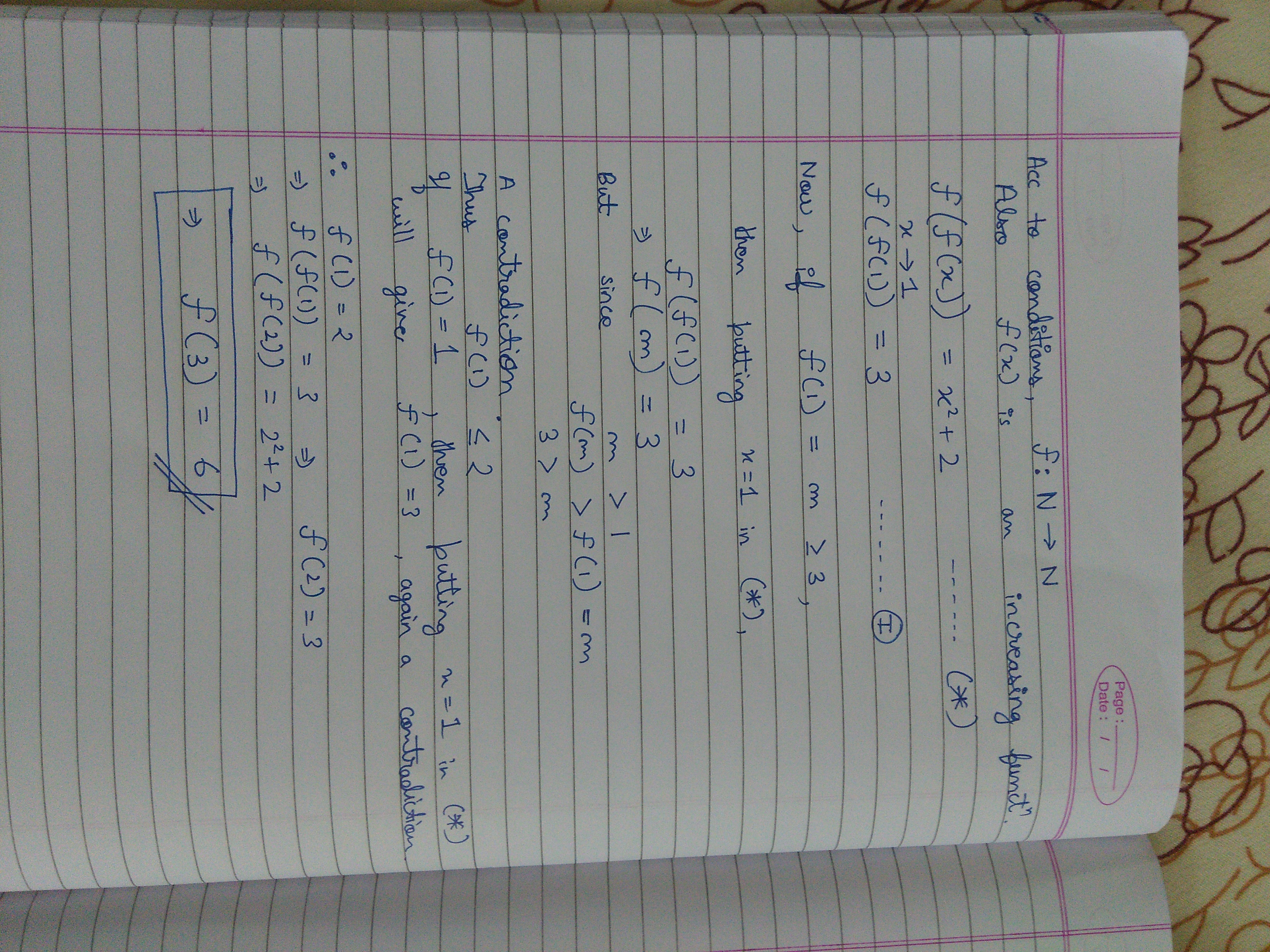- 4 years, 11 months ago

Its right. Post next

- 4 years, 11 months ago

Post next soon

- 4 years, 11 months ago

Problem 4

Let $a,b$ lie in first quadrant and let $x$ be an acute angle.

Given that ($acosx)^2 +(bsinx)^2 = [(sin2x)(\dfrac{a+b}{2} )]^2$

Find the value of tanx.

- 4 years, 11 months ago

If we denote the three vertices by $A(a,b),B(a\csc x,0),C(0,b\sec x)$ then observe that $\displaystyle |AB|^2=a^2\cot^2 x+b^2,|BC|^2=a^2+b^2\tan^2 x,|CA|^2=a^2\csc^2 x +b^2\sec^2 x$

which in turn shows $\displaystyle |AB|^2+|BC|^2=|CA|^2$ and so $\displaystyle B=\frac{\pi}{2}$.

Now, the circumcentre of a right angled triangle lies at the mid-point of it's hypotenuse and so the co-ordinates of the circumcenter is $\displaystyle (M,N)=(\frac{a\csc x}{2},\frac{b\sec x}{2})$

From the relation we have , $\displaystyle a^2\cos^2 x+b^2\sin^2 x=\sin^2 x\cos^2 x(a+b)^2$

$\displaystyle \implies (a\cos^2 x)^2+(b\sin^2 x)^2-2ab\sin^2 x\cos^2 x =0 \implies a\cos^2 x=b\sin^2 x$

So we have , $\displaystyle \csc x = \sqrt{\frac{a+b}{a}} , \sec x=\sqrt{\frac{a+b}{b}}$

So the circumcenter is : $\displaystyle (\frac{\sqrt{a^2+ab}}{2},\frac{\sqrt{b^2+ab}}{2})$

- 4 years, 11 months ago

Correct @Aditya Sharma but sorry I rephrased the problem so @Prince Loomba will decide who will post the next problem.

- 4 years, 11 months ago

- 4 years, 11 months ago

Wait @Harsh Shrivastava , you can't change the problem for the reason someone can't do or have problems with that, the solution is done. Let the problem be back to it's original state

- 4 years, 11 months ago

You post the next prob.

- 4 years, 11 months ago

Post the next

- 4 years, 11 months ago

Circumvented? And is this in the topic list at the starting of note?

- 4 years, 11 months ago

Corrections done.

If I tell the name of the topic, the problem will be trivial :)

- 4 years, 11 months ago

I got $tanx$=$\sqrt {a/b}$. Idk how to find circumcentre

- 4 years, 11 months ago

Lemme rephrase the problem.

- 4 years, 11 months ago

Done post the solution.

- 4 years, 11 months ago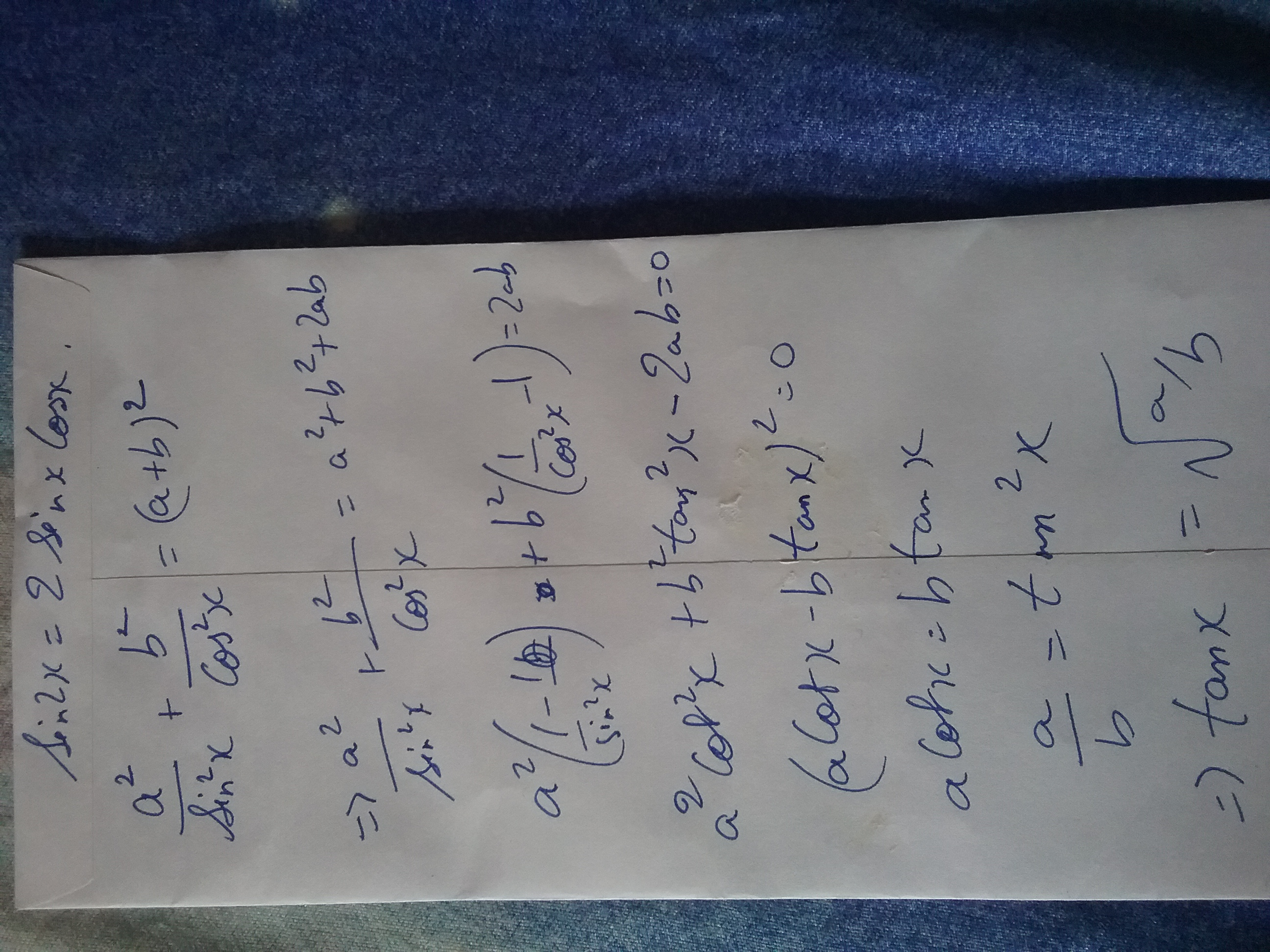- 4 years, 11 months ago

$\text{Problem 5:}$

Find all functions $f:{\rm Z}\to{\rm Z}$ such that $\displaystyle f(m+n)+f(m)f(n)=f(mn+1)$ where $m,n$ are integers.

- 4 years, 11 months ago

Is the answer only 1? (f(x) = 0)

- 4 years, 11 months ago

NO, $f(x)=0$ is trivial, And more functions are there.

- 4 years, 11 months ago

x-1 is also

- 4 years, 11 months ago

Yes one of them, you need to show it other than trial and error.

- 4 years, 11 months ago

I got a weird thing. All of the functions of the type $x^{2n}-1$ are satisfying according to me. Please check.

- 4 years, 11 months ago

hmm ri8 but there are some complicated functions involving mod also. anyways post the solution otherways

- 4 years, 11 months ago

Mod function: (Alternatively the trig function) $f(x) = 0$ if $x\equiv 1, 3 \pmod{4}$, $f(x) = 1$ if $x \equiv 2 \pmod{4}$, $f(x) = -1$ if $x \equiv 0 \pmod{4}$.

- 4 years, 11 months ago

ya those are the solutions exactly. you may post the solution

- 4 years, 11 months ago

I am just saying this. I did not say these are all.

- 4 years, 11 months ago

Two functions f (x)=0 and f (x)=x-1

- 4 years, 11 months ago

There are 6, excluding the zero function

- 4 years, 11 months ago

$x^{2}-1$

- 4 years, 11 months ago

see, dont check your answers then the problem is meaningless . i would suggest that if you hve gor the functions post your solution and i wll cmmnt there

- 4 years, 11 months ago

|x|-1 also satisfies. I am just checking by graph which can hold and verifying my answer

- 4 years, 11 months ago

f(x)=-cos((2n+1)×pi×x/2)

f (x)=sin (n×pi×x)

- 4 years, 11 months ago

Firstly the floor is meaningless here since it's a function from integers to integers. And moreover you are not supposed to guess functions, you have to show the derivation. Otherwise it's not a solution

- 4 years, 11 months ago

One more f (x)={x} fractional part. 6 are done

- 4 years, 11 months ago

f (x)=[x]-1 (floor function)

- 4 years, 11 months ago

There are infinite functions I can make

- 4 years, 11 months ago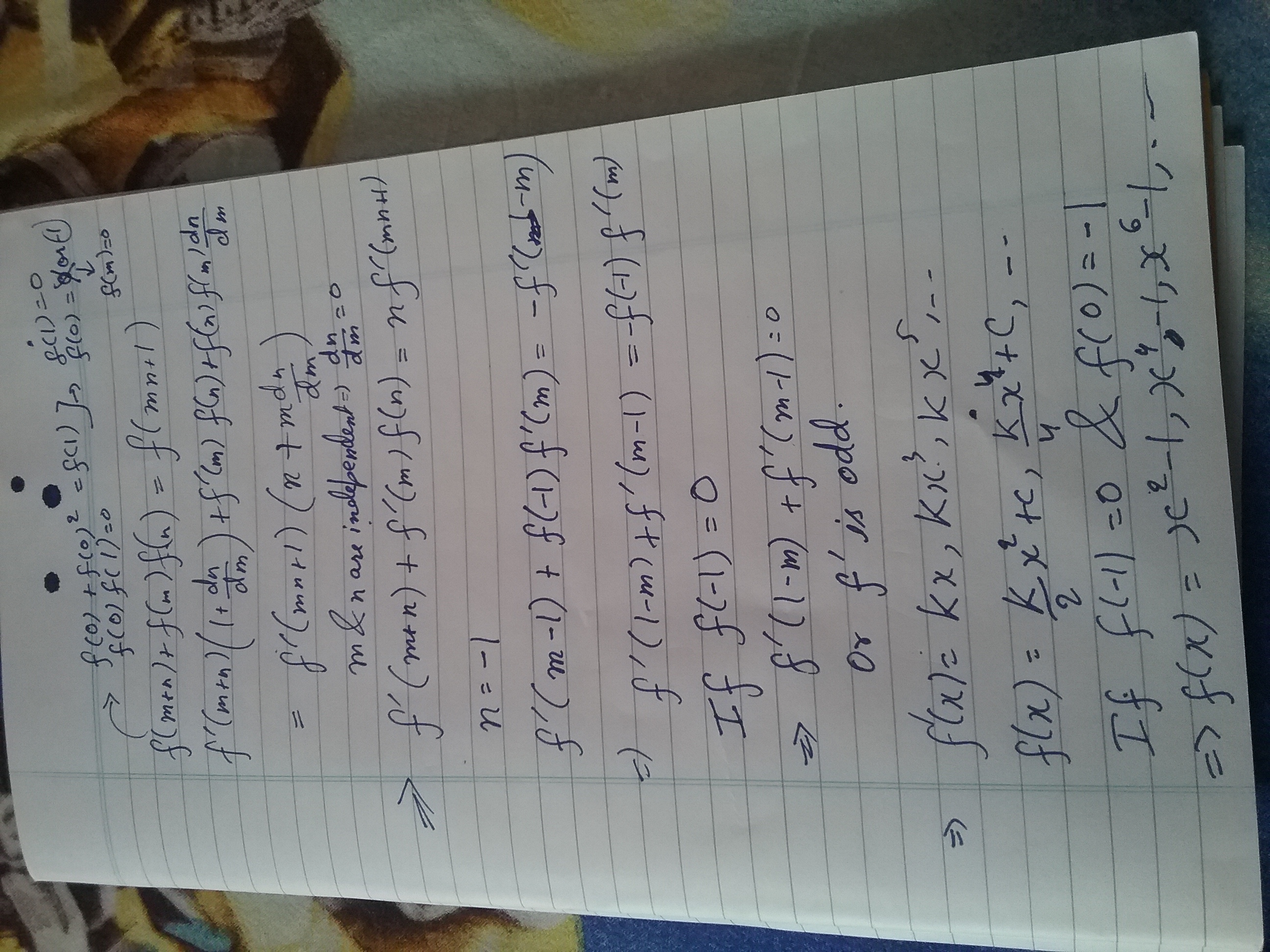- 4 years, 11 months ago

yes it will work , i wll ppst other functions later . for now you may post the next question

- 4 years, 11 months ago

Is there any mistakes I made?

- 4 years, 11 months ago

Problem 26

Can someone please post a solution to my problems!!!

Prove for positive $x$, $y$ and $z$

$\sqrt{x^2+y^2-xy}+\sqrt{y^2+z^2-yz} \geq \sqrt{z^2+x^2+zx}$

- 4 years, 10 months ago

Post easy, I will solve haha

- 4 years, 10 months ago

This is very, very simple. You just have to think outside the box, and you will get it.

- 4 years, 10 months ago

Take a triangle ABC and construct a point P inside the triangle such that angleAPB = angleBPC = angleCPA= 120°.

Now by law of cosines, we can let AP = x,BP = y and CP = z.

The terms in the inequality represents side lengths of triangle ABC and thus the inequality is true by triangle inequality.

- 4 years, 10 months ago

Problem 6

Let $S_{1}, S_{2}, S_{3}$ denote the sum of n terms of 3 arithmetic progressions with first terms unity and their common differences are in harmonic progression. Prove that

$\large n=\frac {2S_{3}S_{1}-S_{1}S_{2}-S_{2}S_{3}}{S_{1}-2S_{2}+S_{3}}.$

- 4 years, 11 months ago

I would like to cut the algebraic bash out of my solution, which I have, but it is quite a standard expansion.

\begin{aligned} S_1 &= \frac {n}{2} (2a+(n-1)d_1)\\ S_2 &= \frac {n}{2} (2a+(n-1)d_2)\\ S_3 &= \frac {n}{2} (2a+(n-1)d_3)\\ d_1 &= \frac{1}{x}\\ d_2 &= \frac {1}{x+k}\\ d_3 &= \frac {1}{x+2k}\\ a &= 1\\ \implies S_1 &= n + \frac{n-1}{2r}\\ S_2 &= n + \frac{n-1}{2r+2k}\\ S_3 &= n + \frac{n-1}{2r+4k} \end{aligned}

Substituting back into the original expression, after much painful expansion, you get the fraction to simplify to $n$.

For those who want the expansion:

\begin{aligned} & \dfrac {2 \left (n + \frac {n-1}{2r} \right ) \left (n + \frac {n-1}{4k+2r} \right ) + \left (n + \frac {n-1}{2r} \right ) \left (n + \frac {n-1}{2r+2k} \right ) + \left (n + \frac {n-1}{2r+2k} \right ) \left (n + \frac {n-1}{2r+4k} \right )}{\left (n + \frac {n-1}{2r} \right ) - 2 \left (n + \frac {n-1}{2r+2k} \right ) + \left (n + \frac {n-1}{2r+4k} \right )}\\ & = \dfrac{2 \left (n + \frac {n-1}{2r} \right ) \left (n + \frac {n-1}{4k+2r} \right ) + \left (n + \frac {n-1}{2r} \right ) \left (n + \frac {n-1}{2r+2k} \right ) + \left (n + \frac {n-1}{2r+2k} \right ) \left (n + \frac {n-1}{2r+4k} \right )}{\frac {k^2 (n-1)}{r(k+r)(2k+r)}}\\ & = \dfrac{r \left ( 2 \left (n + \frac {n-1}{2r} \right ) \left (n + \frac {n-1}{4k+2r} \right ) + \left (n + \frac {n-1}{2r} \right ) \left (n + \frac {n-1}{2r+2k} \right ) + \left (n + \frac {n-1}{2r+2k} \right ) \left (n + \frac {n-1}{2r+4k} \right ) \right )(k+r)(k+2r)}{k^2 (n-1)}\\ &=\dfrac{r \left (\frac {k^2 n(n-1)}{r(k+r)(2k+r)} \right )(k+r)(k+2r)}{k^2 (n-1)}\\ &= \dfrac {k^2 n (n-1)}{k^2 (n-1)}\\ &= n \end{aligned}

- 4 years, 11 months ago

This is not the solution. The solution is 10 times easier. I will consider it as solution either you solve full or take out the shortcut that I have

- 4 years, 11 months ago

Can you please post the easy solution. @Prince Loomba

- 4 years, 11 months ago

Problem 7

Let $H_n = \frac{1}{1} + \frac {1}{2} + \ldots + \frac {1}{n}$. Prove that for $n \geq 2$

$n(n+1)^{\frac {1}{n}} < n + H_n$

- 4 years, 11 months ago

Note that $n+H_n = (1+\frac {1}{1}) + (1+\frac{1}{2}) + \ldots + (1+\frac {1}{n})$ (These brackets are all distinct terms). Now, by AM-GM, we have

\begin{aligned} \dfrac \sum_{i=1}^n \left ( 1+\frac{1}{i} \right ) }{n} &> \sqrt[n]\prod_{i=1}^n \left ( 1+\frac{1}{i} \right )}\\ \dfrac {H_n + n}{n} &> \sqrt[n]{n+1}\\ H_n+n &> n(n+1)^{\frac{1}{n}} \end{aligned

Thus, proven.

- 4 years, 11 months ago

Problem 8

Let $n$ be a positive integer. Prove $(x^2+x)^{2^n} + 1$ is irreducible.

- 4 years, 11 months ago

look at the polynomial mod 2

its equivalent to $(x^{2}+x+1)^{2^{n}}$

so if $(x^{2}+x)^{2^{n}+1}$ $\equiv$ f(x) g(x) mod 2

$f(x)=(x^{2}+x+1)^{m}$ mod 2

$g(x) = (x^{2}+x+1)^{(2^{n}-m)}$ mod 2

$f(x)=(x^{2}+x+1)^{m} + 2P(x)$

$g(x)=(x^{2}+x+1)^{(2^{n}-m)} + 2Q(x)$

$f(x)*g(x)= ((x^{2}+x+1)^m + 2P(x))((x^{2}+x+1)^{(2^n-m)} + 2Q(x))$

Let x=w (complex cube root of unity)

$(w^{2}+w)^{2^{n}} + 1 = 2P(w)*2Q(w)$

2 = 4P(w)Q(w)

We got P (w)Q (w) as rational numbers but they were integer polynomials.

- 4 years, 11 months ago

Let $a\in I$ be a root of it. $(a^{2}+a)^{2^{n}}=-1$. Clearly for n>=1, LHS is a perfect square with range >=0, so inequality cant hold

Thus no integer can be its root.

Hence proved

- 4 years, 11 months ago

Ya same way About to post it But u did it first

- 4 years, 11 months ago

But it is incorrect.

- 4 years, 11 months ago

Yeah U are right.Thanks for explaining

- 4 years, 11 months ago

Not quite. Your method is incorrect since irreducible just means that it cannot be factored. A property of irreducible polynomials (degree > 1) is that they have no integral roots, but the converse isn't true.

- 4 years, 11 months ago

It sufficies to consider the equivalent rational polynomial

$(x^2 -1)^{2^n} + 4^{2^n}$

It is known that every non-trivial binomial coefficient in the rows of Pascal's Triangle which are powers of 2 are all even.

Thus, in the expasion of the above polynomial, only the leading co-efficient and the constant are not even.

The leading coefficient is clearly not divisible by 2.

The constant term is clearly not divisible by 4.

Therefore, Eisenstein's Criterion holds and the polynomial is irreducible.

Hence, the original polynomial is also irreducible.

- 4 years, 11 months ago

Problem 10

Find the number of polynomials of degree 5 with distinct coefficient from the set

{$1,2,...9$}

that are divisible by $x^{2}-x+1$

- 4 years, 11 months ago

Let $P(x)=ax^5 + bx^4 + cx^3 + dx^2 + ex + f$. Note that the roots of $x^2 - x + 1$ are $e^{\frac{i\pi}{3}}$ and $e^{\frac {-i\pi}{3}}$. Thus, $P(x)$ is divisible by $x^2 - x + 1$ if $P(e^{\frac{i\pi}{3}})=0$ and $P(e^{\frac{-i\pi}{3}}) = 0$. From this, we gather that $a+b=d+e$ and $\frac {a}{2} - \frac {b}{2} - c - \frac {d}{2} + \frac {e}{2} + f = 0$, which implies $e + 2f + a = b + 2c + d$. Also, since $a+b=d+e$, $a-d=e-b=c-f$.

We now consider $P(x)= (k+l)x^5 + 9x^4 + (m+l)x^3 + kx^2 + (9+l)x + m$, with $l > 0$ and $k \leq 9 \leq m$. For a given $l$, there are $\dbinom{9-k}{3}$ ways of choosing $k$, $m$ and $n$ such that $l+m \leq 9$. Now since the coefficients are distinct, we must subtract any combination with the difference in $k$, $m$ and $n$ being $l$.

There are $9-2l$ ways to select two numbers differing by $l$ and $7-l$ ways to select the remaining numbers. This implies that for a given $l$, there are $\dbinom{9-l}{3} - (9-2l)(7-l)+9-3l$ polynomials satisfying, which adds up to $53$. However, we take into account the rearrangements of the coefficients (there are 12 for each) so there are $53 \times 12 = 636$ polynomials satisfying.

- 4 years, 11 months ago

Problem 11

Let $f$ be a function $f:\mathbb{Z} \to \mathbb{Z}$ that satisfies below:

$f(y+f(x))=f(x-y)+y(f(y+1)-f(y-1))$

Find all functions that satisfy.

- 4 years, 11 months ago

Put y=0. f (f (x))=f (x)

This implies either f (x) is a constant function or f (x)=x.

- 4 years, 11 months ago

Its a constant function

- 4 years, 11 months ago

And cannot be f(x)=x

- 4 years, 11 months ago

Bro LHS becomes y+f (x)=y+x

RHS becomes x-y+y (y+1-y+1)=x+y

Clearly f (x)=x satisfies

Alternatively take inverse of my equation, you will get f (x)=x

- 4 years, 11 months ago

Sorry calc error

- 4 years, 11 months ago

No problem

- 4 years, 11 months ago

Not necessarily.

- 4 years, 11 months ago

Then you tell. Idk the solutions of f (f(x))=f (x)

- 4 years, 11 months ago

One more: f (x)=|x|, one more f (x)=-|x|

- 4 years, 11 months ago

Here are the solutions:

1) $f(x)=a$ if $x$ is even and $a$ is even, and $f(x)=b$ if $x$ is odd and $b$ is odd.

2) $f (x)=c \forall c$

3)$f (x)=x \forall x$

Sharky discussed some time back this with me so it would be unfair for me to post the solution.

- 4 years, 11 months ago

Well I found these solutions but couldn't find a way to prove that these were the only ones, so I gave up. I'm just waiting for the solution now. (is it some kind of induction?)

- 4 years, 11 months ago

As I have wrote f (f (x))=f (x)

The following satisfy

Constant function

f is odd when x is odd and f is even when x is even

f (x)=x

- 4 years, 11 months ago

Your time is up. 24 hours were given to solve the problem and 2 hours to post the next one for you. 26 hours are over, so by point number 5 since I am creator of problem 10, I can post problem 12!

- 4 years, 11 months ago

Sorry, I was busy, I'll post solution ASAP.

- 4 years, 11 months ago

PROBLEM 12

For the equation $x^{2}+bx+c$, as x approches $\infty$, it also approaches $\infty$.

For the equation $|-x^{2}+bx+c|$, as x approaches $\infty$, it also approaches $\infty$

Can we compare the 2 infinities? If yes, which is greater?

- 4 years, 11 months ago

Yes, we can compare the two infinities. If b is +ve then x^2+bx+c is greater and if b is -ve then |-x^2+bx+c|is greater.

- 4 years, 11 months ago

Right post next

- 4 years, 11 months ago

Problem 13

In problem 12, if c approaches $-\infty$, such that when x tends to $\infty$, $x^{2}+bx+c$ tends to 0.

Which of the following can be the value of $|-x^{2}+bx+c|$?($b\neq 0)$

A) 0

B) $\infty$

C) $-\infty$

D)$\sqrt{2016^{2015}}$

- 4 years, 11 months ago

B

- 4 years, 11 months ago

Wrong. Think again

- 4 years, 11 months ago

B) and D) both can be correct. Check your question again unless it is a multiple options correct question.

- 4 years, 11 months ago

Aman you are right. Post the next. I created this question as multicorrect only!

- 4 years, 11 months ago

And are you guys kidding? Why three contests are going on simultaneously?

- 4 years, 11 months ago

Geometry one is stucked, JEE algebra one I told Rajdeep not to start but he started!

- 4 years, 11 months ago

Your time limit to create a question is up. Read point 5.

- 4 years, 11 months ago

PROBLEM 14

Which of the following sets are incomparable

A). Set of all living children, set of all living humans

B). Set of regular polygons, Set of irregular polygons

C). Set of all the triangles in a plane, set of all the squares in a plane

D). Set of all the things in this universe,set of all the chairs.

Question

You have to explain why all the others are wrong and this is right (Your answer)

- 4 years, 11 months ago

Firstly, in A), we have the the set of all living children is a subset of the set of all living humans, so they can be compared.

Secondly, in C), we have the set of all triangles, which is an uncountable infinite, versus the set of all squares, which is also an uncountable infinite, and we cannot compare these two sets.

Thirdly, in D), we has the set of all chairs is a subset of the set of all things in the universe, so they can be compared.

Finally, in B), we have the set of regular polygons is disjoint from the set of irregular polygons. However, note that the probability an arbitrary polygon is regular is 0 (not impossible, 0), so it is almost guaranteed that an arbitrary polygon is irregular. Thus, we must have the set of all regular polygons to be less than the set of irregular polygons.

- 4 years, 11 months ago

Problem 15

Find the least real number $r$ such that for each triangle with side lengths $a$, $b$, $c$,

$\dfrac {\max(a, b, c)}{\sqrt{a^3 + b^3 + c^3 + 3abc}} < r$

- 4 years, 11 months ago

WLOG, it can be assumed that $\displaystyle a \ge b \ge c$

Using the triangle inequality, and some trivially true statements:

$b + c - a > 0$

$b^2 + c^2 + a^2 + ab + ac - bc > 0$

$b^3 + c^3 - a^3 + 3abc > 0$

$a^3 + b^3 + c^3 + 3abc > 2a^3$

The rest should follow after rearranging...

$\dfrac {a^3}{a^3+b^3+c^3+3abc} < \dfrac {1}{2}$

$\dfrac {a}{\sqrt{a^3+b^3+c^3+3abc}} < \dfrac {1}{\sqrt{2}}$

Thus, $\displaystyle r=\frac {1}{\sqrt{2}}$.

- 4 years, 11 months ago

Problem 16

Evaluate: $\displaystyle \sum_{n=0}^{\infty} \cos^n{\theta} \cos{n \theta}$

Assume θ is such that the sum converges.

- 4 years, 11 months ago

is it ? i replaced theta with x $\frac12.\frac{2e^{ix}-\cos x - \cos x e^{2ix}}{\cos^2 x e^{ix} - \cos x - \cos x e^{2ix}+e^{ix}}$

- 4 years, 11 months ago

It is assumed that I want the answer in the simplest possible form...

- 4 years, 11 months ago

I will prove the fraction simplifies to 2. We are required to prove:

\begin{aligned} \dfrac{2e^{ix}-\cos x - \cos x e^{2ix}}{\cos^2 x e^{ix} - \cos x - \cos x e^{2ix}+e^{ix}}&= 2\\ 2e^{ix} - \cos x - \cos x e^{2ix} &= 2\cos^2 x e^{ix} - 2\cos x - 2\cos x e^{2ix} + 2e^{ix}\\ \cos x e^{2ix} + \cos x &= 2 \cos^2 x e^{ix}\\ \cos x (2 \cos^2 x + 2i \sin x \cos x) &= 2 \cos^2 x (\cos x + i \sin x)\\ 2 \cos^2 x (\cos x + i \sin x) &= 2 \cos^2 x (\cos x + i \sin x) \end{aligned}

which is clearly true. Thus, the original fraction must be equal to 2, so the value of the summation is 1.

- 4 years, 11 months ago

Problem 18

Since @Archit Agrawal's time ran out, I'll post next question.

Consider the sequence

$a_n = 2 - \dfrac {1}{n^2 + \sqrt{n^4 + \frac {1}{4}}}, \quad n \geq 1$

Prove that $\sqrt{a_1}+\sqrt{a_2}+\ldots+\sqrt{a_{119}}$ is an integer and find the value of it.

- 4 years, 11 months ago

$\boxed{168}$

- 4 years, 11 months ago

\begin{aligned} a_n &= 2 - \dfrac {1}{n^2 + \sqrt{n^4+\frac {1}{4}}}\\ &= 2 - \dfrac{n^2 - \sqrt{n^4 + \frac {1}{4}}}{n^4 - \left ( n^4 + \frac {1}{4} \right )}\\ &= 2 + 4n^2 - 2 \sqrt{4n^4 + 1} \end{aligned}